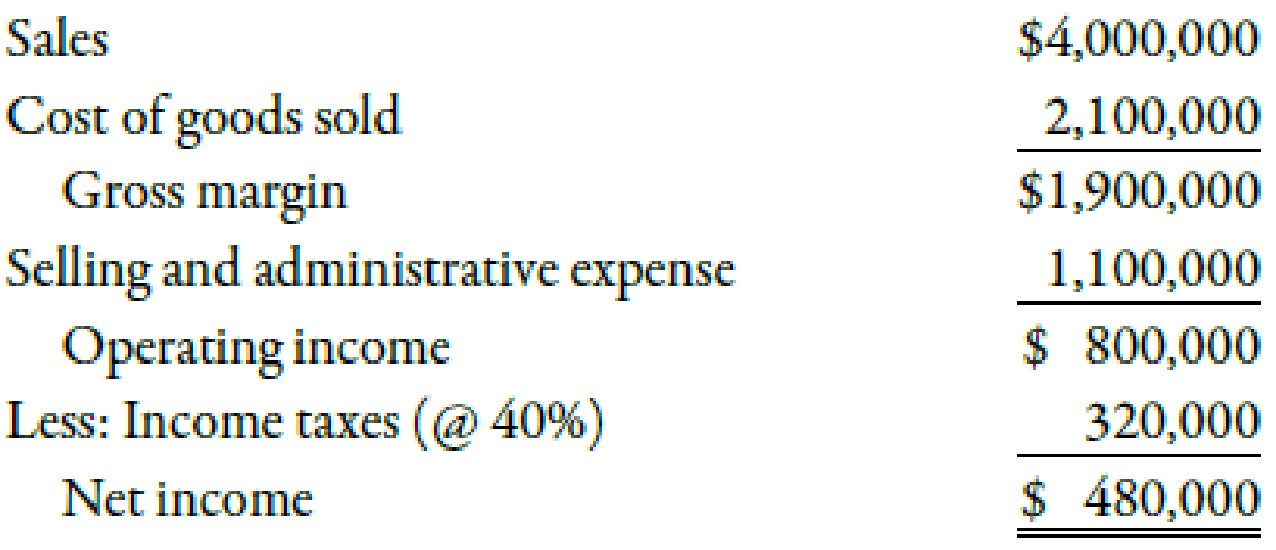Chapter 11, Problem 21BEB### Managerial Accounting: The Corners...

7th Edition
Maryanne M. Mowen + 2 others
ISBN: 9781337115773

#### Solutions

Chapter
Section### Managerial Accounting: The Corners...

7th Edition
Maryanne M. Mowen + 2 others
ISBN: 9781337115773
Textbook Problem
13 views

# Use the following information for Brief Exercises 11-19 through 11-21:Barnard Manufacturing earned operating income last year as shown in the following income statement:At the beginning of the year, the value of operating assets was $2,700,000. At the end of the year, the value of operating assets was$2,300,000.Brief Exercise 11-21 Calculating Economic Value AddedRefer to the information for Barnard Manufacturing on the previous page. Total capital employed equaled $1,400,000. Barnard’s actual cost of capital is 12%.Required:Calculate the EVA for Barnard Manufacturing. To determine Compute EVA for B Manufacturing. Explanation Economic Value Added (EVA): Economic value added can be computed by deducting the dollar amount of capital employed from the operating income after tax. The dollar amount of capital employed can be calculated by multiplying the capital employed by the percentage of the cost of capital. Use the following formula to calculate the economic value added: EVA=After tax operating income(Total capital employed×Actual percentage of cost of capital) Substitute$480,000 for after-tax operating income, \$1,400,000 for total capital employed and 12% for the actual percentage of the cost of capital in the above formula

### Still sussing out bartleby?

Check out a sample textbook solution.

See a sample solution

#### The Solution to Your Study Problems

Bartleby provides explanations to thousands of textbook problems written by our experts, many with advanced degrees!

Get Started